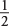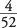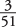## Probability of Joint Occurrences

Another way to compute the probability of all three flipped coins landing heads is as a series of three different events: First flip the penny, then flip the nickel, and then flip the dime. Will the probability of landing three heads still be 0.125?

#### Multiplication rule

To compute the probability of joint occurrence (two or more independent events all occurring), multiply their probabilities.

For example, the probability of the penny landing heads is, or 0.5; the probability of the nickel next landing heads is, or 0.5; and the probability of the dime landing heads is, or 0.5. Thus, note that

0.5 × 0.5 × 0.5 = 0.125

which is what you determined with the classic theory by assessing the ratio of the number of favorable outcomes to the number of total outcomes. The notation for joint occurrence is

P( AB) = P( A) × P( B)

which is read: The probability of A and B both happening is equal to the probability of A times the probability of B.

Using the multiplication rule, you also can determine the probability of drawing two aces in a row from a deck of cards. The only way to draw two aces in a row from a deck of cards is for both draws to be favorable. For the first draw, the probability of a favorable outcome is. But because the first draw is favorable, only three aces are left among 51 cards. So, the probability of a favorable outcome on the second draw is. For both events to happen, you simply multiply those two probabilities together:Note that these probabilities are not independent. If, however, you had decided to return the initial card drawn back to the deck before the second draw, then the probability of drawing an ace on each draw is, because these events are now independent. Drawing an ace twice in a row, with the odds beingboth times, gives the following:In either case, you use the multiplication rule because you are computing probability for favorable outcomes in all events.

Given mutually exclusive events, finding the probability of at least one of them occurring is accomplished by adding their probabilities.

For example, what is the probability of one coin flip resulting in at least one head or at least one tail?

The probability of one coin flip landing heads is 0.5, and the probability of one coin flip landing tails is 0.5. Are these two outcomes mutually exclusive in one coin flip? Yes, they are. You cannot have a coin land both heads and tails in one coin flip; therefore, you can determine the probability of at least one head or one tail resulting from one flip by adding the two probabilities:

0.5 + 0.5 = 1 (or certainty)

##### Example 1
What is the probability of at least one spade or one club being randomly chosen in one draw from a deck of cards?

The probability of drawing a spade in one draw is; the probability of drawing a club in one draw is. These two outcomes are mutually exclusive in one draw because you cannot draw both a spade and a club in one draw; therefore, you can use the addition rule to determine the probability of drawing at least one spade or one club in one draw: